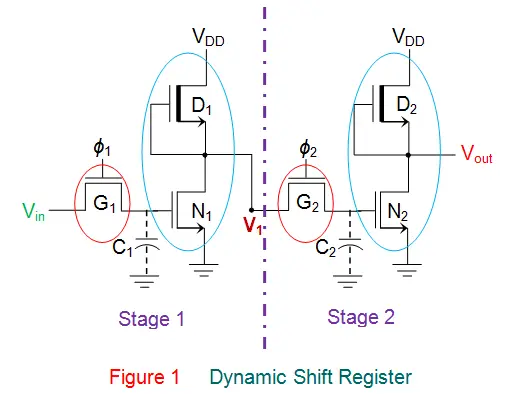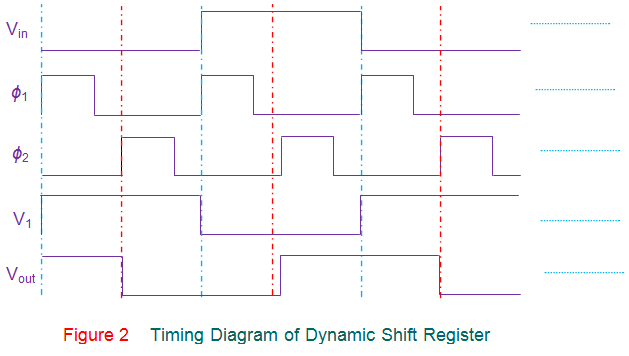# Dynamic Shift Register

Shift registers can be classified into two types viz., Static Shift Registers and Dynamic Shift Registers. Static shift registers are composed of flip-flops and are capable of storing the information within them for indefinite period of time. On the other hand, dynamic shift registers comprise of dynamic inverters and employ temporary charge storage techniques and hence require frequent refresh cycles to store the data.
Figure 1 shows a dynamic shift register formed by a combination of NMOS transmission gates (red circled components, G1 and G2) and NMOS depletion-mode inverters (blue circled components, {D1, N1} and {D2, N2}). Here ϕ1 and ϕ2 are non-overlapping, mutually complementary clock signals, while C1 and C2 represent the gate-to-source capacitances of Stage 1 and Stage 2, respectively. Further these capacitances are considered to be depleted of charge in their initial state.Now consider Vin = 0V and ϕ1 = VDD, which correspond to the logic states 0 and 1 respectively. For this case, the gate G1 will be open (non-conducting) and thus the capacitor C1 will remain in its uncharged state.

This causes the output voltage level of the inverter circuit in Stage 1 (formed by D1 and N1) to go high i.e. V1 = VDD (assuming zero threshold voltage for all the devices in the circuit – just for simplicity). However for this, ϕ1 is to be maintained in its high state for sufficient amount of time, as the charging of the capacitor is a gradual process (considering RC time constant issue). Now if ϕ2 goes high then the gate G2 closes due to which the capacitor C2 starts to charge through it gradually to the voltage level of VDD (= V1), imposing the restrictions on the clock frequency in use. Further it is to be noted that as the voltage across the capacitor C2 increases, the output voltage at the Stage 2 decreases due to the inverting action of the circuit formed by formed by D2 and N2. This in turn causes the output voltage, Vout to go low (= 0V). Hence it can be said that the state of Vin is shifted to Vout.

Similarly if Vin = VDD while ϕ1 = VDD, then C1 charges to VDD through G1 causing the output voltage of Stage 1, V1 to go low. At this instant if ϕ2 = VDD, then gate G2 closes, the capacitor C2 discharges while the output voltage, Vout increases gradually. Thus one gets Vout = VDD, reflecting the logic high state of input voltage. Hence, once again, the state of Vin is reflected at Vout, which implies that the Vin is shifted to Vout under the control of the clock. This means that the circuit shown in Figure 1 acts as a single stage shift register. However an n-stage dynamic shift register can be designed by cascading n number of such stages.

The working of the single stage dynamic shift register can be further emphasized by the timing diagram shown by Figure 2.From the explanation, it is clear that the dynamic shift registers store the information in the form of charge on the gate-to-substrate parasitic capacitance of the electronic (especially MOS) devices. However this charge is prone to leakage and thus one requires refreshing the data periodically in order to ensure that the logic levels of the data stored are error-free. This objective is achieved by continuously shifting the data from one stage to another while feeding the output of the last stage back to the first stage. This means that dynamic shift registers must be operated at the minimum clock frequency.
Dynamic shift registers are simpler in terms of fabrication and have high package density due to their smaller size. However it is to be noted that their advantage of less power consumption is cursed by the fact that the power consumed increases with the increase in frequency. Further there are many design variations available in case of dynamic shift registers like dynamic shift registers using enhancement load, dynamic shift registers using CMOS devices and so on including Ratioed Logic approach as well as Ratio less Logic approach. Nevertheless, the basic working principle remains the same.

Want To Learn Faster? 🎓
Get electrical articles delivered to your inbox every week.
No credit card required—it’s 100% free.